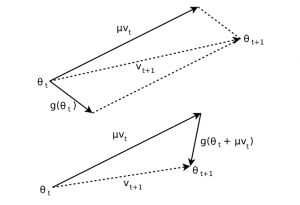/  Deep Learning Interview questions and answers   /  What is the difference between Momentum and Nesterov Momentum?## What is the difference between Momentum and Nesterov Momentum?

Ans: Momentum method is a technique that can speed up gradient descent by taking accounts of previous gradients in the update rule at every iteration.where v is the velocity term, the direction and speed at which the parameter should be twisted and α is the decaying hyper-parameter, which determines how quickly collected previous gradients will decay. If α is much bigger than η, the accumulated previous gradients will be dominant in the update rule so the gradient at the iteration will not change the current direction quickly. In the other hand, if α is much smaller than η, the accumulated previous gradients can act as a smoothing factor for the gradient.

The difference between Momentum method and Nesterov Accelerated Gradient is the gradient computation phase. In Momentum method, the gradient was calculated using current parameters θ𝑡whereas in Nesterov Accelerated Gradient, we apply the velocity vt to the parameters θ to calculate interim parameters θ̃ . We then calculate the gradient using the interim parametersAfter we get the gradients, we update the parameters using similar update rule with the Momentum method (Eq. 2), with the difference in the gradientsWe can consider Nesterov Accelerated Gradients as the correction factor for Momentum method.Fig. 2 (Top) Momentum method, (Bottom) Nesterov Accelerated Gradient. 𝜇 is the decaying parameter, same as α in our case (Sutskever et al., 2013, Figure 2)

When the learning rate η is relatively large, Nesterov Accelerated Gradients allows larger decay rate α than Momentum method, while preventing oscillations. Both Momentum method and Nesterov Accelerated Gradient become equivalent when η is small.